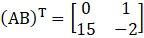# RD Sharma Solutions For Class 12 Maths Exercise 5.4 Chapter 5 Algebra of Matrices

RD Sharma Solutions for Class 12 Maths Exercise 5.4 Chapter 5 Algebra of Matrices is provided here for students to study and score good marks in the board exams. In order to excel in the CBSE exam for Class 12, students can use the solutions of Exercise 5.4 available on the website. By practising regularly the students can obtain worthy results in the Class 12 exams by using the RD Sharma Solutions.

The PDF of RD Sharma Solutions of exercise 5.4 primarily helps students from the exam point of view. This exercise mainly contains solved problems based on the concept of symmetric and skew-symmetric matrices. RD Sharma Solutions for Class 12 Maths Chapter 5 Algebra of Matrices Exercise 5.4 are provided here.

## Download the PDF of RD Sharma Solutions For Class 12 Chapter 5 – Algebra of matrices Exercise 5.4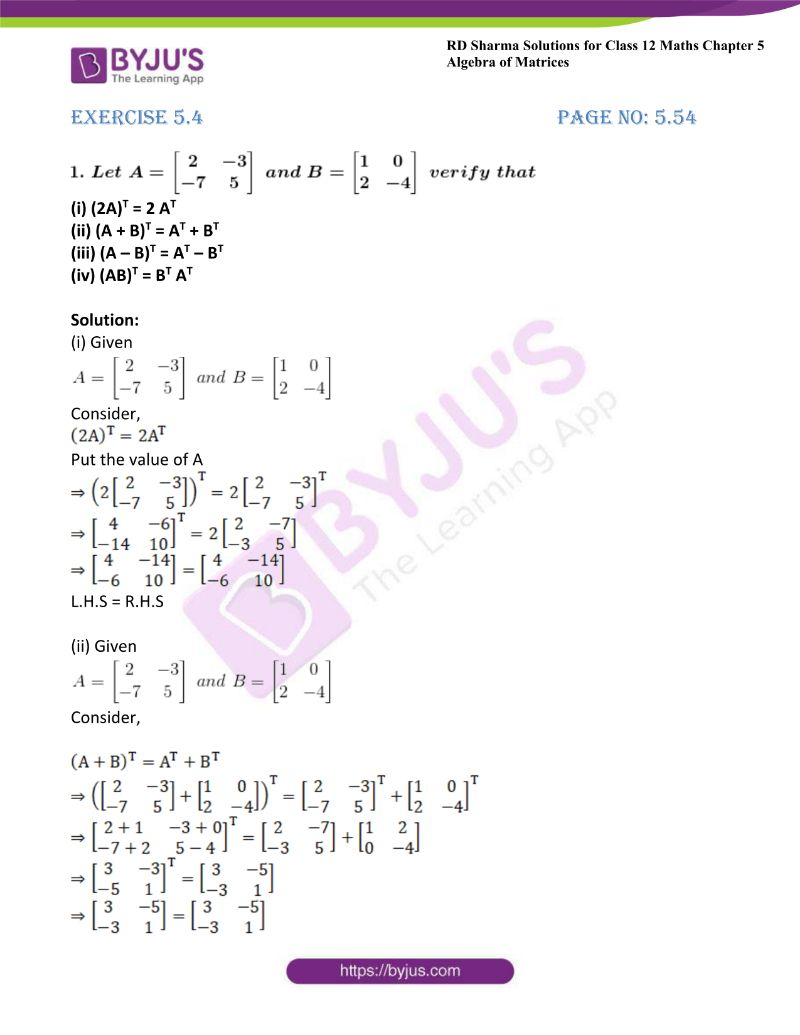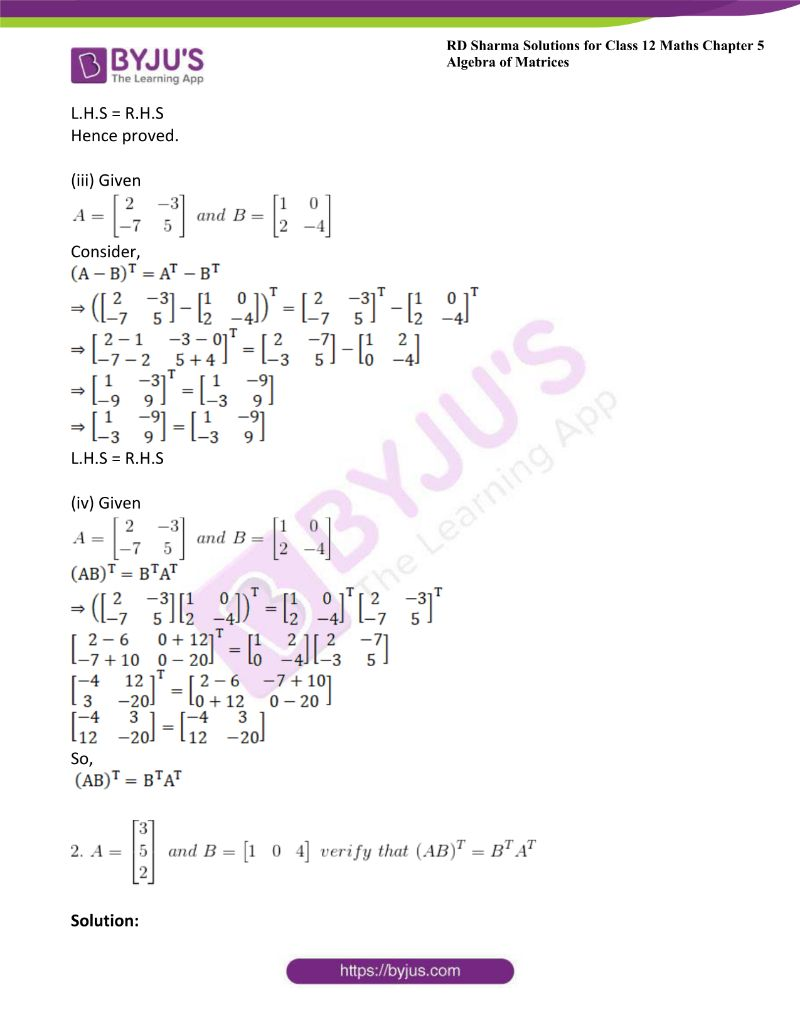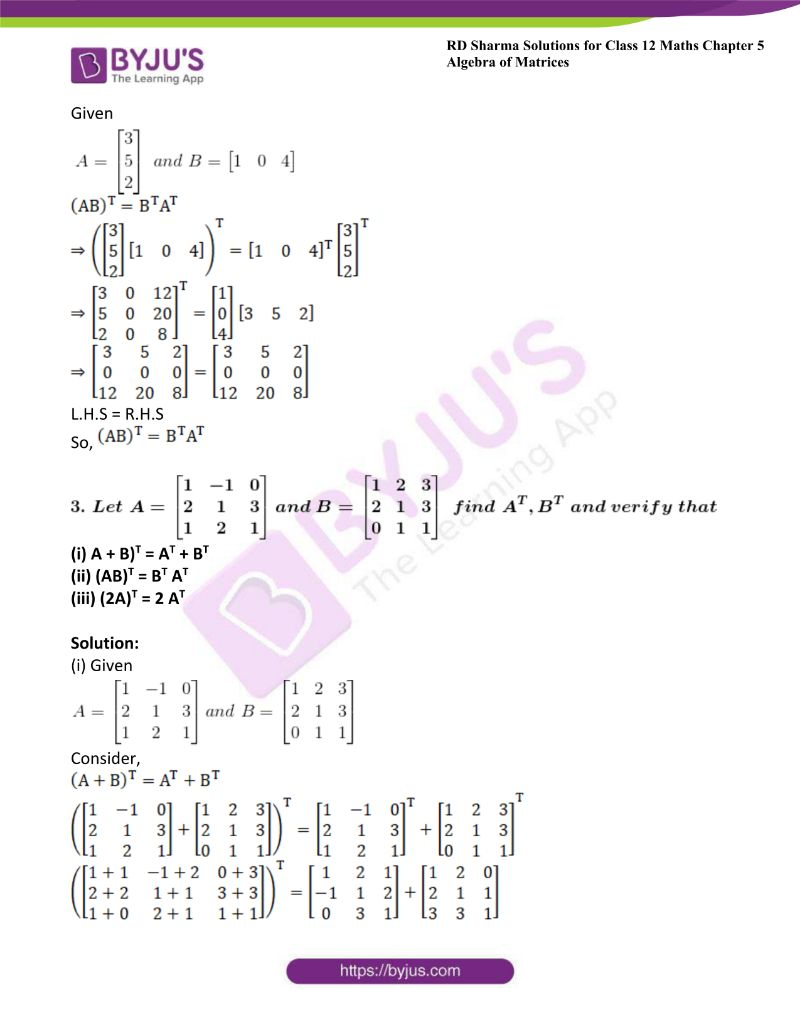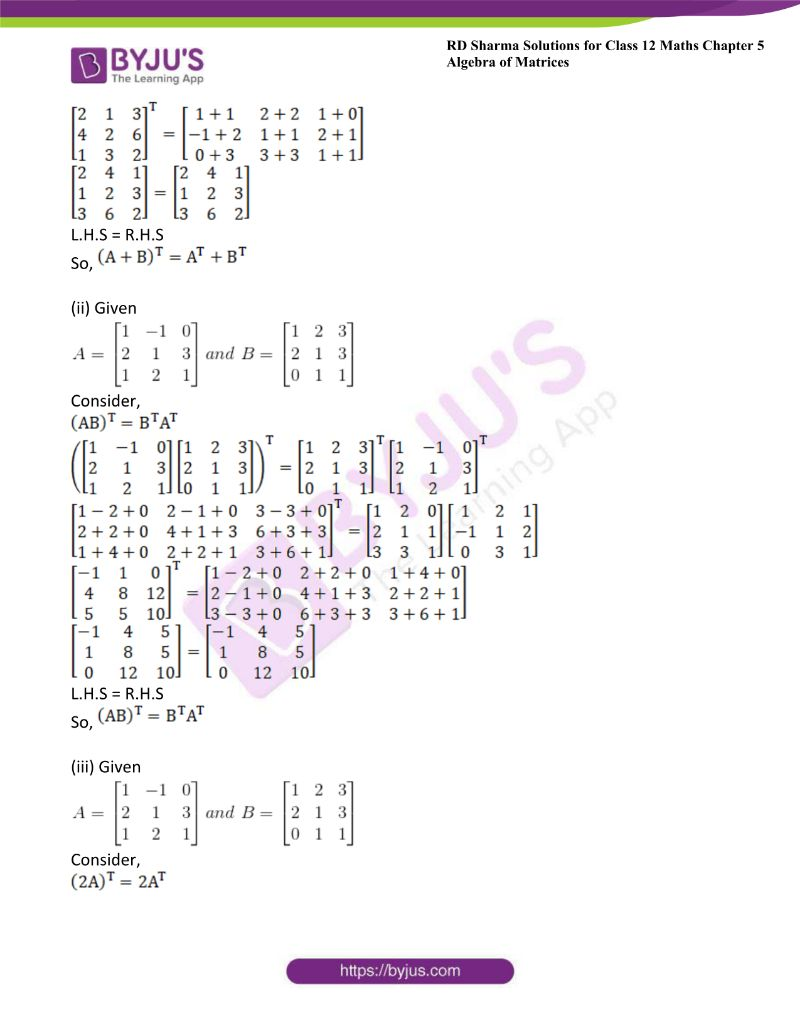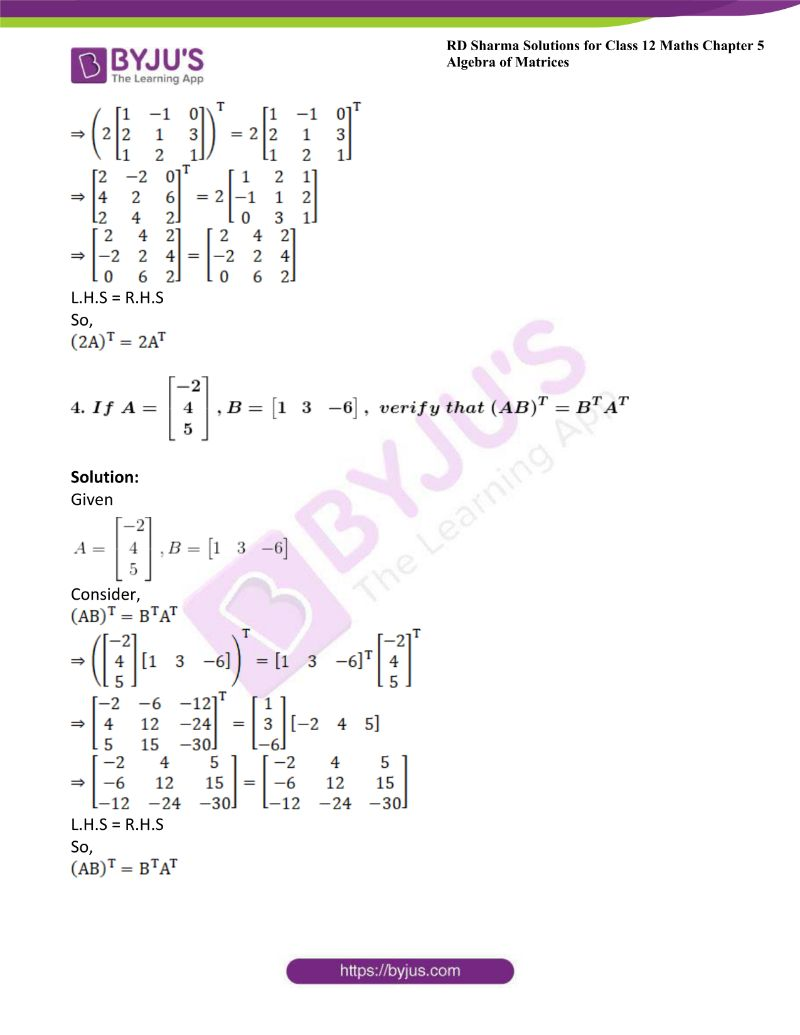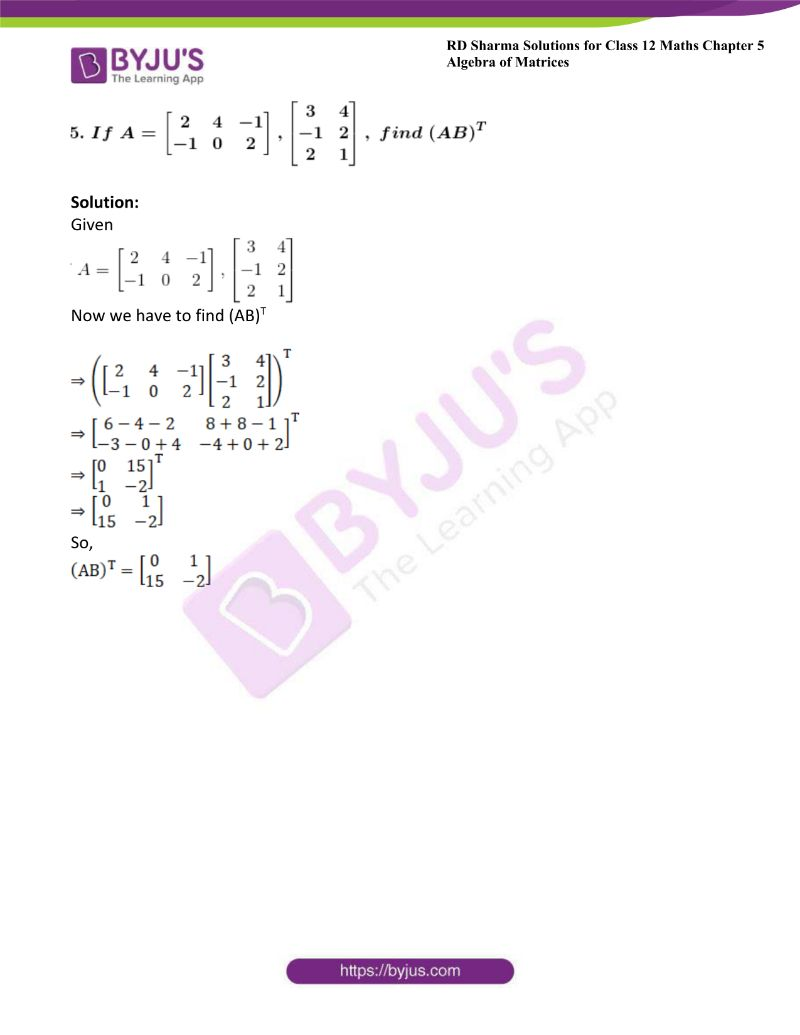### Access other exercises of RD Sharma Solutions For Class 12 Chapter 5 – Algebra of Matrices

Exercise 5.1 Solutions

Exercise 5.2 Solutions

Exercise 5.3 Solutions

Exercise 5.5 Solutions

### Access answers to Maths RD Sharma Solutions For Class 12 Chapter 5 – Algebra of Matrices Exercise 5.4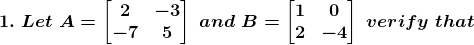(i) (2A)T = 2 AT

(ii) (A + B)T = AT + BT

(iii) (A – B)T = AT – BT

(iv) (AB)T = BT AT

Solution:

(i) Given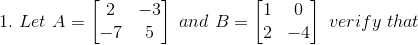Consider,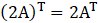Put the value of A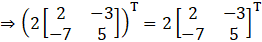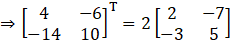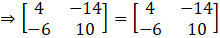L.H.S = R.H.S

(ii) GivenConsider,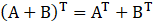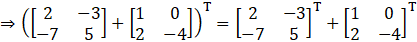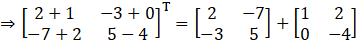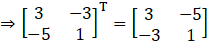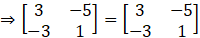L.H.S = R.H.S

Hence proved.

(iii) GivenConsider,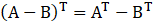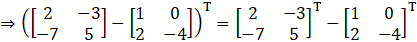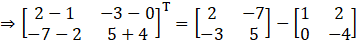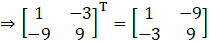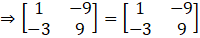L.H.S = R.H.S

(iv) Given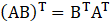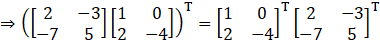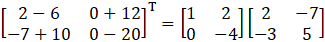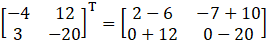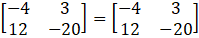So,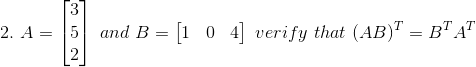Solution:

Given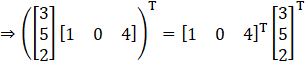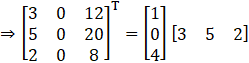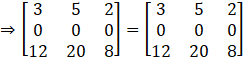L.H.S = R.H.S

So,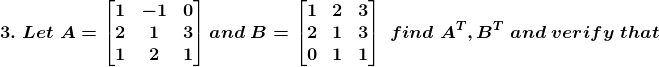(i) A + B)T = AT + BT

(ii) (AB)T = BT AT

(iii) (2A)T = 2 AT

Solution:

(i) Given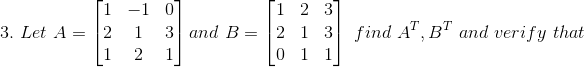Consider,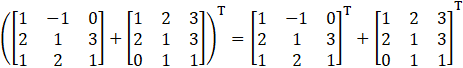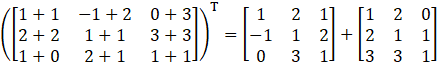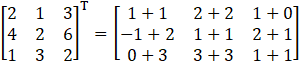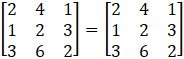L.H.S = R.H.S

So,(ii) GivenConsider,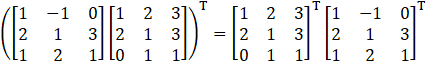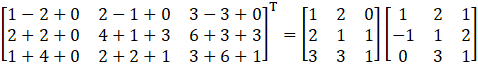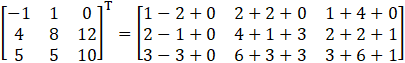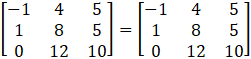L.H.S = R.H.S

So,(iii) GivenConsider,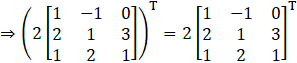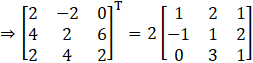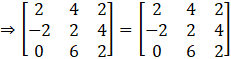L.H.S = R.H.S

So,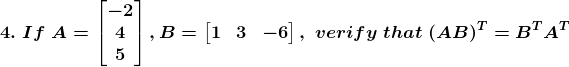Solution:

Given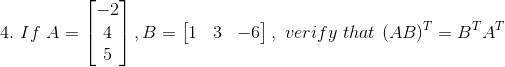Consider,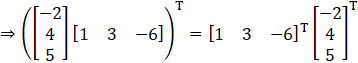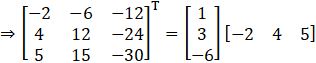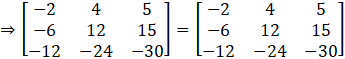L.H.S = R.H.S

So,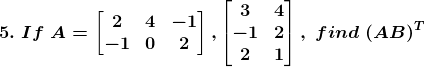Solution:

Given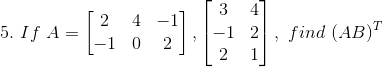Now we have to find (AB)T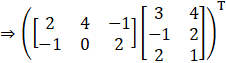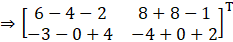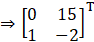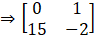So,